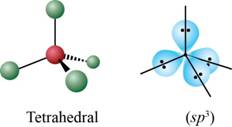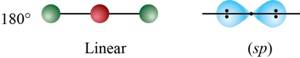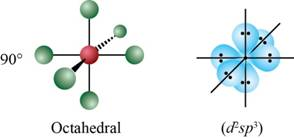# Explain the main postulate of the VSEPR model. List the five base geometries (along with bond angles) that most molecules or ions adopt to minimize electron-pair repulsions. Why are bond angles sometimes slightly less than predicted in actual molecules as compared to what is predicted by the VSEPR model?### Chemistry: An Atoms First Approach

2nd Edition
Steven S. Zumdahl + 1 other
Publisher: Cengage Learning
ISBN: 9781305079243### Chemistry: An Atoms First Approach

2nd Edition
Steven S. Zumdahl + 1 other
Publisher: Cengage Learning
ISBN: 9781305079243

#### Solutions

Chapter 4, Problem 1RQ
Textbook Problem

## Explain the main postulate of the VSEPR model. List the five base geometries (along with bond angles) that most molecules or ions adopt to minimize electron-pair repulsions. Why are bond angles sometimes slightly less than predicted in actual molecules as compared to what is predicted by the VSEPR model?

Expert Solution
Interpretation Introduction

Interpretation: The main postulates of VSEPR theory are to be stated and the five base geometries that most molecules adopt to minimize electron-pair repulsion are to be predicted. An explanation corresponding to the bond angles being slightly less than predicted in case of actual molecules as compared to what are predicted by the VSEPR theory is to be stated.

Concept introduction: Valance shell electron pair repulsion theory commonly called as vsepr theory. It is used to predict the geometry of molecules from the number of electron pairs surrounding their central atom. The five geometries are described that most molecules adopt to minimize electron-pair repulsion.

To determine: The postulates of VSEPR model; the five base geometries that most molecules adopt to minimize electron-pair repulsion and an explanation corresponding to the bond angles being slightly less than predicted in actual molecules, as compared to what is predicted by the VSEPR theory.

### Explanation of Solution

Explanation

The main postulates of VSEPR theory are stated as follows.

The main postulates of VSEPR theory are,

• The shape of the molecule is determined by the repulsion between all the electron pairs.
• A lone pair of electron occupies more space than bond pair.
• The electrons are oriented around the central atom in such a way that the repulsion among them is minimum
• The order of repulsion among various electron pairs is,

Lone pairLone pair> Lone pairBond pair >Bond pair Bond pair

• The magnitude of repulsion between bond pair of electrons depend upon the electronegativity difference between central atom and bonded atoms
• Triple bonds cause more repulsion than double bonds. Similarly double bonds cause more repulsion than single bonds

The five base geometries are stated as follows.

The five base geometries that most molecules adopt to minimize electron-pair repulsion are,

• Tetrahedral (Bond angle 109°28' )
•• Figure 1

• Trigonal planar (Bond angle 120° )Figure 2

• Linear (Bond angle 180° )Figure 3

• Trigonal bipyramidal (Bond angle 90° , 120° )Figure 4

• Octahedral (Bond angle 90° )Figure 5

Due to the repulsion between the lone pair and the bond pair, the bond angle is sometimes different than that predicted by the VSPER theory

The bond angles are sometimes slightly less than predicted in actual molecules as compared to that predicted by VSPER theory because in the above mentioned geometries, the bond angle varies with the presence of a lone pair.

Due to the repulsion between the lone pair and the bond pair the geometry of the molecule changes from the original shape of the given molecule.

Conclusion

Conclusion

The main postulates of VSEPR theory have been rightfully stated.

Due to the repulsion between the lone pair and the bond pair the geometry of the molecule changes from the original shape of the given molecule

### Want to see more full solutions like this?

Subscribe now to access step-by-step solutions to millions of textbook problems written by subject matter experts!

### Want to see more full solutions like this?

Subscribe now to access step-by-step solutions to millions of textbook problems written by subject matter experts!